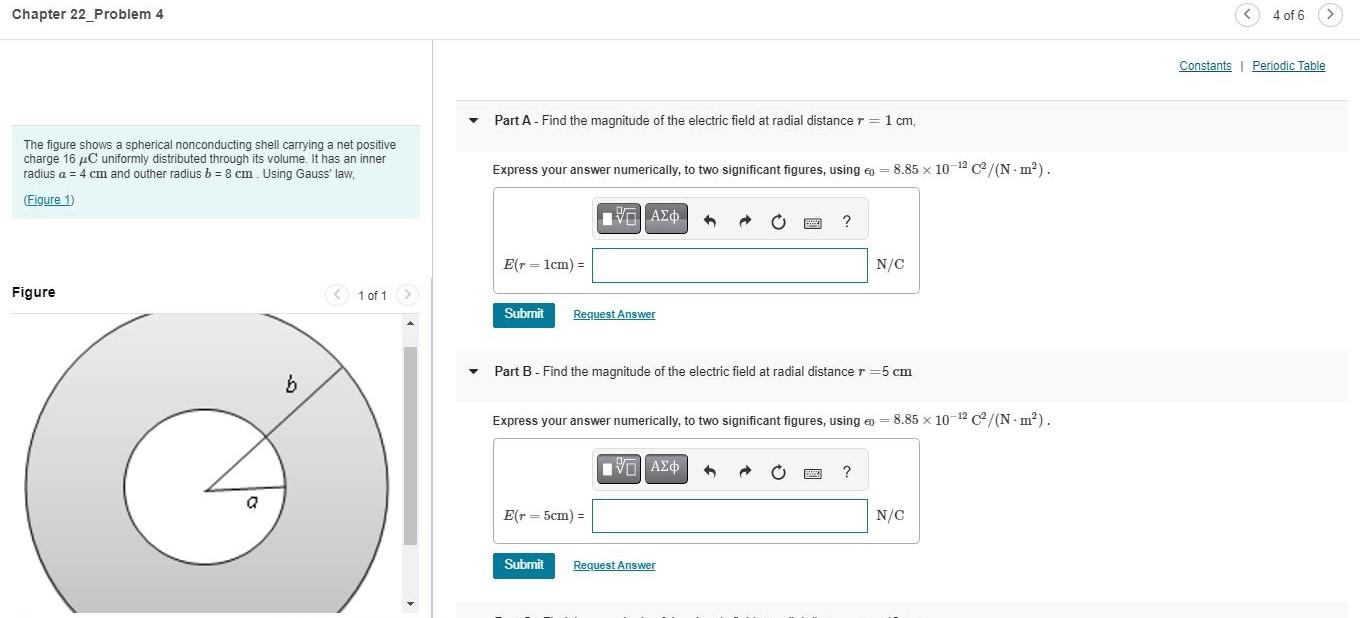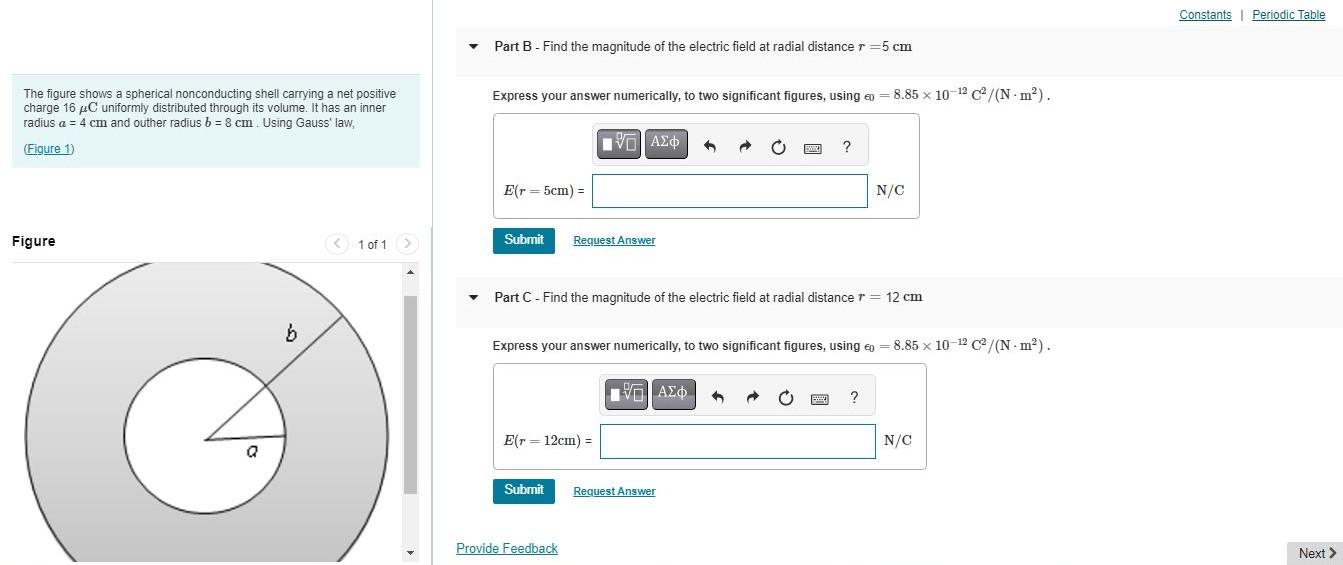# Question Part A - Find the magnitude of the electric field at radial distance $$r=1 \mathrm{~cm}$$, The figure shows a spherical nonconducting shell carrying a net positive charge $$16 \mu \mathrm{C}$$ uniformly distributed through its volume. It has an inner radius $$a=4 \mathrm{~cm}$$ and outher radius $$b=8 \mathrm{~cm}$$. Using Gauss' law Express your answer numerically, to two significant figures, using $$\epsilon_{0}=8.85 \times 10^{-12} \mathrm{C}^{2} /\left(\mathrm{N} \cdot \mathrm{m}^{2}\right)$$. (Figure 1) Part B - Find the magnitude of the electric field at radial distance $$r=5 \mathrm{~cm}$$ Express your answer numerically, to two significant figures, using $$\epsilon 0=8.85 \times 10^{-12} \mathrm{C}^{2} /\left(\mathrm{N} \cdot \mathrm{m}^{2}\right)$$. Part B - Find the magnitude of the electric field at radial distance $$r=5 \mathrm{~cm}$$ The figure shows a spherical nonconducting shell carrying a net positive charge $$16 \mu \mathrm{C}$$ uniformly distributed through its volume. It has an inner radius $$a=4 \mathrm{~cm}$$ and outher radius $$b=8 \mathrm{~cm}$$. Using Gauss' law, (Figure 1) Part C - Find the magnitude of the electric field at radial distance $$r=12 \mathrm{~cm}$$ Express your answer numerically, to two significant figures, using $$\epsilon_{0}=8.85 \times 10^{-12} \mathrm{C}^{2} /\left(\mathrm{N} \cdot \mathrm{m}^{2}\right)$$.Transcribed Image Text: Part A - Find the magnitude of the electric field at radial distance $$r=1 \mathrm{~cm}$$, The figure shows a spherical nonconducting shell carrying a net positive charge $$16 \mu \mathrm{C}$$ uniformly distributed through its volume. It has an inner radius $$a=4 \mathrm{~cm}$$ and outher radius $$b=8 \mathrm{~cm}$$. Using Gauss' law Express your answer numerically, to two significant figures, using $$\epsilon_{0}=8.85 \times 10^{-12} \mathrm{C}^{2} /\left(\mathrm{N} \cdot \mathrm{m}^{2}\right)$$. (Figure 1) Part B - Find the magnitude of the electric field at radial distance $$r=5 \mathrm{~cm}$$ Express your answer numerically, to two significant figures, using $$\epsilon 0=8.85 \times 10^{-12} \mathrm{C}^{2} /\left(\mathrm{N} \cdot \mathrm{m}^{2}\right)$$. Part B - Find the magnitude of the electric field at radial distance $$r=5 \mathrm{~cm}$$ The figure shows a spherical nonconducting shell carrying a net positive charge $$16 \mu \mathrm{C}$$ uniformly distributed through its volume. It has an inner radius $$a=4 \mathrm{~cm}$$ and outher radius $$b=8 \mathrm{~cm}$$. Using Gauss' law, (Figure 1) Part C - Find the magnitude of the electric field at radial distance $$r=12 \mathrm{~cm}$$ Express your answer numerically, to two significant figures, using $$\epsilon_{0}=8.85 \times 10^{-12} \mathrm{C}^{2} /\left(\mathrm{N} \cdot \mathrm{m}^{2}\right)$$.
Transcribed Image Text: Part A - Find the magnitude of the electric field at radial distance $$r=1 \mathrm{~cm}$$, The figure shows a spherical nonconducting shell carrying a net positive charge $$16 \mu \mathrm{C}$$ uniformly distributed through its volume. It has an inner radius $$a=4 \mathrm{~cm}$$ and outher radius $$b=8 \mathrm{~cm}$$. Using Gauss' law Express your answer numerically, to two significant figures, using $$\epsilon_{0}=8.85 \times 10^{-12} \mathrm{C}^{2} /\left(\mathrm{N} \cdot \mathrm{m}^{2}\right)$$. (Figure 1) Part B - Find the magnitude of the electric field at radial distance $$r=5 \mathrm{~cm}$$ Express your answer numerically, to two significant figures, using $$\epsilon 0=8.85 \times 10^{-12} \mathrm{C}^{2} /\left(\mathrm{N} \cdot \mathrm{m}^{2}\right)$$. Part B - Find the magnitude of the electric field at radial distance $$r=5 \mathrm{~cm}$$ The figure shows a spherical nonconducting shell carrying a net positive charge $$16 \mu \mathrm{C}$$ uniformly distributed through its volume. It has an inner radius $$a=4 \mathrm{~cm}$$ and outher radius $$b=8 \mathrm{~cm}$$. Using Gauss' law, (Figure 1) Part C - Find the magnitude of the electric field at radial distance $$r=12 \mathrm{~cm}$$ Express your answer numerically, to two significant figures, using $$\epsilon_{0}=8.85 \times 10^{-12} \mathrm{C}^{2} /\left(\mathrm{N} \cdot \mathrm{m}^{2}\right)$$.&#12304;General guidance&#12305;The answer provided below has been developed in a clear step by step manner.Step1/4It is given that,the charge stored in the shell is $$\mathrm{{q}={16}~\mu{C}}$$the inner radius of the shell is $$\mathrm{{a}={4}~{c}{m}={0.04}~{m}}$$the outer radius of the shell is $$\mathrm{{b}={8}~{c}{m}={0.08}~{m}}$$It is required to find the magnitude of the electric field at the radial distance of,a) $$\mathrm{{r}={1}~{c}{m}}$$b) $$\mathrm{{r}={5}~{c}{m}}$$c) $$\mathrm{{r}={12}~{c}{m}}$$Explanation:To use Gauss's law to find the electric field at a distance $$\mathrm{{r}}$$ from the center of the spherical shell, we need to choose a Gaussian surface that encloses the charge distribution. Since the charge is uniformly distributed throughout the volume of the shell, a natural choice is a spherical Gaussian surface with radius $$\mathrm{{r}}$$.We can then apply Gauss's law, which states that the electric flux through any closed surface is proportional to the charge enclosed by the surface:$$\mathrm{\oint{\mathbf{{{E}}}}\cdot{d}{\mathbf{{{A}}}}={\frac{{{Q}_{{{e}{n}{c}}}}}{{\epsilon_{{0}}}}}~~~~~~~~~~~~~{\left({1}\right)}}$$where $$\mathrm{{\mathbf{{{E}}}}}$$ is the electric field, $$\mathrm{{d}{\mathbf{{{A}}}}}$$ is an infinitesimal area element on the Gaussian surface, $$\mathrm{{Q}_{{{e}{n}{c}}}}$$ is the charge enclosed by the surface, and $$\mathrm{\epsilon_{{0}}}$$ is the permittivity of free space.Since the charge distribution is spherically symmetric, the electric field must also be spherically symmetric. Therefore, we can choose the Gaussian surface to be a sphere of radius $$\mathrm{{r}}$$, centered at the center of the shell. The electric f ... See the full answer